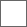• 16回复贴，共1

### 【评分楼】2019年第八届MW杯小组赛第四题评分楼

A.设计分

B.游戏性分

C.加分与扣分

A.设计分

B.游戏性分

C.加分与扣分

D3选手 小皓宇Tom 小组赛第四题关卡最终得分：（93.4+93）/2=93.2

A.设计分

B.游戏性分

C.加分与扣分

A.设计分

B.游戏性分

C.加分与扣分

C2选手 把僵尸炖了 小组赛第四题关卡最终得分：（96.5+96.8）/2=96.65≈96.7

A.设计分

B.游戏性分

C.加分与扣分

A.设计分

B.游戏性分

C.加分与扣分

D1选手 878yfy 小组赛第四题关卡最终得分：（83.3+81）/2=82.15≈82.2A.设计分

B.游戏性分

C.加分与扣分

A.设计分

B.游戏性分

C.加分与扣分

D2选手 suteaury 小组赛第四题关卡最终得分：（87.9+89.1）/2=88.5

A.设计分

B.游戏性分

C.加分与扣分

A、设计分

B、游戏性分

C、加分与扣分

C3选手 绿色的糖果233 小组赛第四题关卡最终得分：（60+54）/2=57A.设计分

B.游戏性分

C.加分与扣分

A.设计分

B.游戏性分

C.加分与扣分

A3选手 王一凡01234567 小组赛第四题参赛关卡最终得分：（61.7+68.9）/2=65.3

A.设计分

B.游戏性分

C.加分与扣分

A.设计分

B.游戏性分

C.加分与扣分

A2选手 2333ty 小组赛第四题参赛关卡最终得分：（73.1+75）/2=74.05≈74.1

A.设计分

B.游戏性分

C.加分与扣分

A.设计分

B.游戏性分

C.加分与扣分

A1 koopa4 小组赛第四题参赛关卡最终得分：（87.2+91.9）/2=89.55≈89.6

A.设计分

B.游戏性分

C.加分与扣分

A.设计分

B.游戏

C.加分与扣分

B1选手 1168438795 小组赛第四题参赛关卡最终得分：（95+96.2）/2=95.6

A.设计分

B.游戏性

C.加分与扣分

A.设计分

B.游戏性

C.加分与扣分

B2选手 s小s飞s侠s 小组赛第四题最终得分：（94.5+95.5）/2=95

A.设计分

B.游戏性分

C.加分与扣分

A.设计分

B.游戏性

C.加分与扣分

B3选手 我懂你不懂的lz 小组赛第四题最终得分：（96.5+98.3）/2=97.4

A.设计分

B.游戏性分

C.加分与扣分

A.设计分

B.游戏性

C.加分与扣分

B4选手 数字1528君 小组赛第四题最终得分：（92.5+94）/2=93.25≈93.3

#### 扫二维码下载贴吧客户端